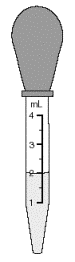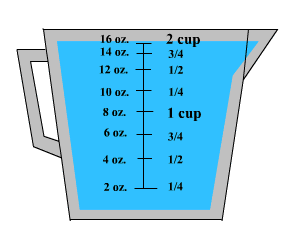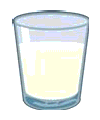# Capacity

Capacity refers to the amount that can be held in a container. Capacity can be measured in metric units or in U.S. customary units.

## Metric Units

The standard metric unit of capacity is the liter.

From the liter, we get the rest of the metric units using the standard metric prefixes.

 Centiliters(cl) $10$ milliliters (ml) Deciliters (dl) $10$ centiliters or $100$ milliliters Liter (l) $10$ deciliters or $1,000$ milliliters Dekaliter (dal) $10$ liters Hectoliter (hl) $10$ dekaliters or $100$ liters Kiloliter (kl) $10$ hectoliters or $1,000$ litersThe eye dropper in the figure has a $2$ ml of liquid.

## U.S. Customary Units

The system for measuring capacity in the customary system is based on cup, fluid ounces, pint, quart and gallon.

In the figure shown, $2$ cups of water is equal to $1$ pint.The most commonly used customary units of capacity are shown.

 Unit Model $1$ fluid ounce (fl oz) $2$ tablespoons of water $1$ cup (c) = $8$ fluid ounce (fl oz ) coffee cup $1$ pint (pt) = $2$ cups small ice cream container $1$ quart (qt) = $2$ pints large liquid measuring cup $1$ gallon (gal) = $4$ quarts large plastic jug of milk

To convert from one unit to another, we can use unit analysis . For Example, a glass of milk is greater than an eyedropper and less than a small pitcher. Then estimate the capacity of glass of milk.We know that, $1$ liter = $1,000$ milliliters.

A small pitcher can fill about $4$ glasses.

One estimate for the capacity of a glass of milk is about $1,000÷4$ or $250$ milliliters.

Note:

$\left(\text{a}\right)$ To convert from larger units to smaller units, multiply by the appropriate unit ratio.

$\left(\text{b}\right)$ To convert from smaller units to larger units, multiply by the reciprocal of the appropriate unit ratio.

$\left(\text{c}\right)$ Multiplying or dividing by a unit ratio is mathematically equivalent to using a proportion to convert between units of measure .